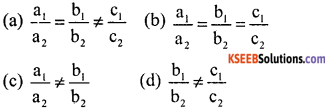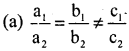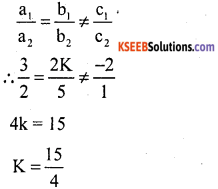# KSEEB Solutions for Class 10 Maths Chapter 3 Pair of Linear Equations in Two Variables Additional Questions

Students can Download Class 10 Maths Chapter 3 Pair of Linear Equations in Two Variables Additional Questions Questions, Notes Pdf, KSEEB Solutions for Class 10 Maths helps you to revise the complete Karnataka State Board Syllabus and score more marks in your in examinations

## Karnataka State Syllabus Class 10 Maths Chapter 3 Pair of Linear Equations in Two Variables Additional Questions

I. Multiple Choice Questions:

Question 1.
Which of the following is not a linear equation
(a) x + 3y = 8
(b) x = 2y
(c) x2 + 5x + 8 = 0
(d) y = 0.
(c) x2 + 5x + 8 = 0

Question 2.
x = 1 and y = 3 is a solution of a linear equation.
(a) x – y = 4
(b) x + y = 4
(c) 2x + y = 8
(d) 2y + x = 9
(b) x + y = 4

Question 3.
The pair of co-ordinates satisfying 2x + y = 6 is
(a) (2, 2)
(b) (2, 3)
(c) (4, 1)
(d) (5, 1)
(a) (2, 2)Question 4.
The pair of equations x = 0 and y = 0 represents.
(a) Parallel lines
(b) Coincident lines
(c) non-intersecting lines
(d) Perpendicular intersecting lines
(d) Perpendicular intersecting lines

Question 5.
If the ten’s and unit’s digits of a two digit number are y and x respectively, then the number will be.
(a) 10y + x
(b) 10x + y
(c) 10xy
(d) y + x
(a) 10y + x

Question 6.
If the line y = px – 2 passes through the point (2, 2), then the value of P is
(a) 3
(b) 2
(c) 5
(d) 4
(b) 2

Question 7.
The coordinates of the origin are.
(a) (0, 1)
(b) (1, 0)
(c) (2, 2)
(d) (0, 0)
(d) (0, 0)

Question 8.
The point (-3, -4) lies in the Quadrant.
(a) I
(b) II
(c) III
(d) IV
(c) III

Question 9.
The point of intersection of the lines x – 2 – 0 and y + 6 = 0 is
(a) (0, 0)
(b) (0, -6)
(c) (0, 2)
(d) (2, -6)
(d) (2, -6)Question 10.
The pair of equations x = 4 and y = 3 graphically represents lines which are
(a) Parallel
(b) intersecting at (3, 4)
(c) Coincident
(d) intersecting at (4, 3)
(d) intersecting at (4, 3)

Question 11.
Two lines are given to be parallel the equation of one of the lines is 4x + 3y = 14. The equation of the second line can be.
(a) 3x + 4y =14
(b) 8x + 6y = 28
(c) – 12x = 9y
(d) 12x + 9y = 42
(c) – 12x = 9y

Question 12.
The pair of equations x + y = 0 and x + y = – 7 has
(a) one solution
(b) no solution
(c) two solutions
(d) infinitely many solutions
(b) no solution

Question 13.
The pair of linear equations 3x + 5y = 3 and 6x + Ky = 8 does not have a solution if.
(a) K = 5
(b) K ≠ 10
(c) K = 10
(d) K ≠ 5.
(c) K = 10

Question 14.
If y = 2x – 3 and y = 5 then the value of x is.
(a) 1
(b) 2
(c) 3
(d) 4
(d) 4Question 15.
The sum of two numbers is 8 and their difference is 2. Find the numbers.
(a) 5 and 3
(b) 6 and 4
(c) 4 and 2
(d) 4 and 4
(a) 5 and 3

Question 16.
The larger of two supplementary angles exceeds the smaller by 18 degrees. Find them.
(a) 98°, 82°
(b) 99°, 81°
(c) 118, 100°
(d) 80°, 100°
(b) 99°, 81°

Question 17.
The value of a so that the point (3, a) lies on the line represented by 2x – 3y = 5 is
(a) 1/3
(b) – 1/3
(c) 1
(d) 2/3
(b) – 1/3

Question 18.
If the pair of linear equations a1 x + b, y + c, = 0 and a2x + b2y + c2 = 0 has a unique solution, then.Question 19.
The solution of the pair of equations x – y = 0, 2x – y = 0 is
(a) x = 3, y = 5
(b) x = 3, y = 2
(c) x = 2, y = 2
(d) x = 5, y = 5
(c) x = 2, y = 2Question 20.
The value of x if y = 1/2x and 3x + 4y = 20 is
(a) 3
(b) 5
(c) 6
(d) 4
(d) 4

Question 1.
Find the coordinate where the line x – y = 8 will intersect the y-axis.
The given line will intersect y-axis when x = 0 ∴ 0 – y = 8
y = – 8

Question 2.
If the lines given by 3x + 2ky = 2 and 2x + 5y + 1 = 0 are parallel, then find value of k.
Since given lines are parallelQuestion 3.
Write the number of solutions of the following pair of linear equations.